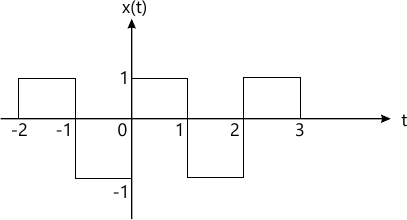MORE IN Signals & Systems
Total marks: --
Total time: --
INSTRUCTIONS
(1) Assume appropriate data and state your reasons
(2) Marks are given to the right of every question
(3) Draw neat diagrams wherever necessary

Answer any one question from Q1 and Q2
1 (a) Perform the following operations on the given signal x(t) which is defined as:
x(t) = u(t) u(t-4)
i) Sketch z(t)=x(-t -1)
ii) Sketch y(t)=x(t)+ z(t).
4 M
1 (b) Determine whether the following signals are Energy or Power, and find energy or time averaged power of the signal:
i) x(t)=5 cos (πt) + sin (5πt); - ∞ ≤ t ≤ ∞
 ii) x[n] =n, 0 ≤ n ≤ 5 = 10 -n, 5 ≤ n ≤ 10 = 0, otherwise
4 M
1 (c) Determine whether the following system in Static/Dynamic, Causal/Non-causal and Stable/Unstable and justify:
h(t)=e-5t u(t).
4 M

2 (a) Compute the convolution integral by graphical method and sketch the output for the following signals:
x(t)=u(t)-u(t-2)
h(t)=e-2t u(t)
6 M
2 (b) Evaluate the following integrals: $i) \ \ \int^\infty _0 t^2 \delta (t-10)dt \\ ii) \ \int^{10}_0 \delta (t) \sin 2 (\pi t) dt$
4 M
2 (c) Determine whether the following signal is periodic or not, if periodic, find the fundamental period of the signal:
x(t)=cos2 (2π t).
2 M

Answer any one question from Q3 and Q4
3 (a) Find the trigonometric Fourier series for the periodic signal x(t) shown in the following figure and sketch the amplitude and phase spectra:6 M
3 (b) Find the inverse Laplace transform of:
$X(s) = \dfrac {2} {(s+4)(s-1)}$ If the Region of convergence is:
i) -4 ≤ Re(s) <1
ii) Re(s) > 1
iii) Re(s) < - 4.
6 M

4 (a) Find the Fourier transform of the following signals:
i) x(t) = sng(t)
ii) x(t)=u(t)
iii) x(t)=e-at sin (ω0 t) u(t).
6 M
4 (b) Find the initial and final value of the following signal: $X(s) = \dfrac { 2s+3} {s^2 + 5s -7}$
4 M
4 (c) State the relationship between Fourier transform and Laplace transform.
2 M

Answer any one question from Q5 and Q6
5 (a) Find the following for the given signal x(t):
i) Autocorrelation
ii) Energy from Autocorrelation
iii) Energy Spectral Density.
x(t) e-10 u(t).
6 M
5 (b) Determine the cross-correlation between two sequence which are given below:
x1(n)={1 2 3 4}
x2(n) = {3 2 1 0}
4 M
5 (c) State and describe any three properties of Power Spectral Density (PSD).
3 M

6 (a) Prove that autocorrelation function and energy spectral density from Fourier transform pair of each other and verify the same for:
x(t) e-10 u(t).
9 M
6 (b) State and describe any four properties of Energy Spectral Density (ESD).
4 M

Answer any one question from Q7 and Q8
7 (a) Explain Exponential probability model with respect to its density and distribution function.
4 M
7 (b) Two cards are drawn from a 52 card deck successively without replacing the first:
i) Given the first one is heart, what is the probability that second is also a heart?
ii) What is the probability that both cards will be hearts?
4 M
7 (c) A coin is tossed three times. Write the sample space which gives all possible outcomes. A random variable X, which represents the number of heads obtained on any double toss. Draw the mapping of S on to real line. Also find the probabilities of X and plot the C.D.F.
5 M

8 (a) PDF of a random variable X is: fx(x)= ke-10x, x>0 and
fx(x)=0 x≥0.
Find: i) value of k
ii) P(1 ≤ X ≤ 2)
iii) P(X ≥ 3).
6 M
8 (b) State the properties of Cumulative probability distribution function.
3 M
8 (b) Find the mean standard deviation and variance of the uniform random variable.
4 M

More question papers from Signals & Systems